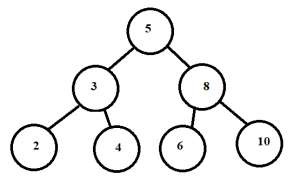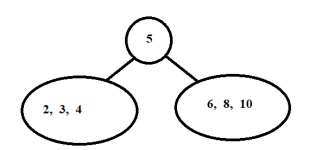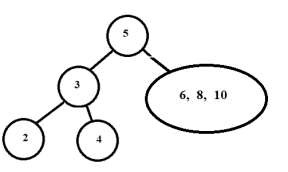# Construct Tree from given Inorder and Preorder traversals in C++

We are given the Inorder and Preorder traversals of a binary tree. The goal is to construct a tree from given traversals.

Inorder traversal − In this type of tree traversal, a left subtree is visited first, followed by the node and right subtree in the end.

Inorder (tree root)

• Traverse left subtree of node pointed by root, call inorder ( root→left )

• Visit the root

• Traverse right subtree of node pointed by root, call inorder ( root→right )

Preorder traversal − In this type of tree traversal, the node visited first, followed by the left subtree and right subtree in the end.

Preorder (tree root)

• Visit the root
• Traverse left subtree of node pointed by root, call inorder ( root→left )
• Traverse right subtree of node pointed by root, call inorder ( root→right )

The inorder and preorder traversal of below tree are given −## Inorder

2-3-4-5-6-8-10

## Preorder

4-3-2-5-8-6-10

Now we’ll construct the above tree again for given preorder and inorder traversals.

## Inorder

2-3-4-5-6-8-10

## Preorder

5-3-2-4-8-6-10
• As we know that preorder visits the root node first then the first value always represents the root of the tree. From above sequence 5 is the root of the tree.

## Preorder

5 -3-2-4-8-6-10
• From above inorder traversal, we know that a node’s left subtree is traversed before it followed by its right subtree. Therefore, all values to the left of 5 in inorder belong to its left subtree and all values to the right belong to its right subtree.

## Inorder

2-3-4 ← 5 → 6-8-10• Now for the left subtree do the same as above.

Preorder traversal of left subtree is 3 -2-4. So 3 becomes the root.

Inorder traversal divided further into 2 ← 3 → 4• Now for the right subtree do the same as above.

Preorder traversal of the right subtree is 8 -6-10. So 8 becomes the root.

Inorder traversal divided further into 6 ← 8 → 10So, in this way we constructed the original tree from given preorder and inorder traversals.